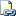# LEFT (Transact-SQL)

Returns the left part of a character string with the specified number of characters.Transact-SQL Syntax Conventions

## Syntax

``````LEFT ( character_expression , integer_expression )
``````

## Arguments

• character_expression
Is an expression of character or binary data. character_expression can be a constant, variable, or column. character_expression can be of any data type, except text or ntext, that can be implicitly converted to varchar or nvarchar. Otherwise, use the CAST function to explicitly convert character_expression.

• integer_expression
Is a positive integer that specifies how many characters of the character_expression will be returned. If integer_expression is negative, an error is returned. If integer_expression is type bigint and contains a large value, character_expression must be of a large data type such as varchar(max).

The integer_expression parameter counts a UTF-16 surrogate character as one character.

## Return Types

Returns varchar when character_expression is a non-Unicode character data type.

Returns nvarchar when character_expression is a Unicode character data type.

## Remarks

When using SC collations, the integer_expression parameter counts a UTF-16 surrogate pair as one character. For more information, see Collation and Unicode Support.

## Examples

### A. Using LEFT with a column

The following example returns the five leftmost characters of each product name.

``````USE AdventureWorks2012;
GO
SELECT LEFT(Name, 5)
FROM Production.Product
ORDER BY ProductID;
GO
``````

### B. Using LEFT with a character string

The following example uses LEFT to return the two leftmost characters of the character string abcdefg.

``````SELECT LEFT('abcdefg',2)
GO
``````

Here is the result set.

``````--
ab

(1 row(s) affected)
``````